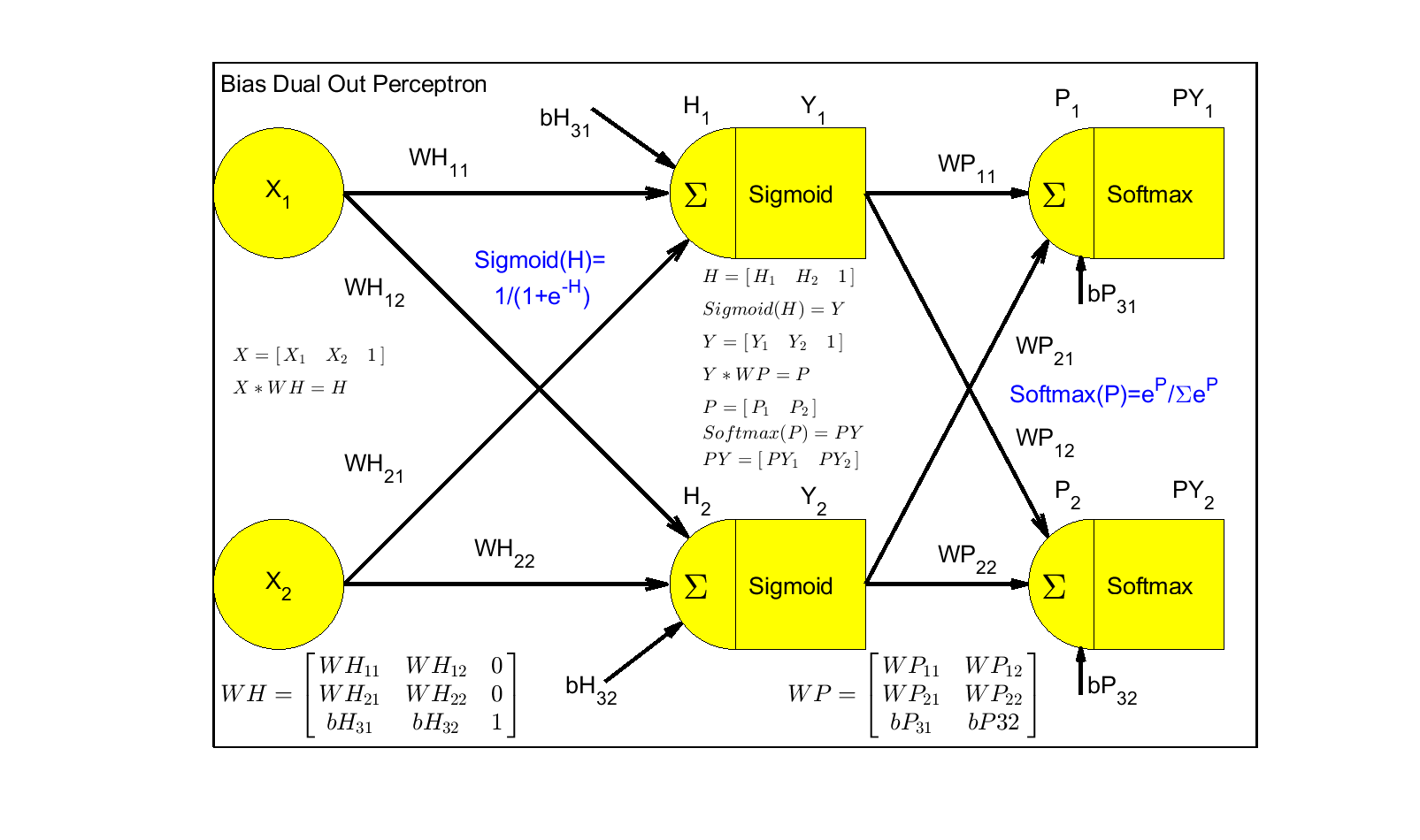# Problem 58902. Neural Net: Calculate Bias Dual Output Perceptron (AND/NAND/OR/NOR/XOR/XNOR)

This challenge is to calculate the Neural Net Bias Dual Output Perceptron vector,PY and Pclass, given X, WH, and WP using Sigmoid on the hidden layer and Softmax on the output layer. Test Cases will be all two input conditions for functions XOR, XNOR, AND, NAND, OR, and NOR.
X wll be a 4x3 matrix creating a Dual output PY 4x2 matrix. PY(:,1) is Expectation of 0 and PY(:,2) is Expectation of 1 for each case. PY values shall all be <0.25 or >.75 The Pclass must match the Logical Function expectation where Pclass is the column of PY with max value per row, col1=Logic0 and col2=Logic1.
[PY,Pclass]=Calc_Dual_Bias_Perceptron(X,WH,WP)The function template includes the code to create this figure using Matlab Latex

### Solution Stats

80.0% Correct | 20.0% Incorrect
Last Solution submitted on Sep 25, 2023

### Community Treasure Hunt

Find the treasures in MATLAB Central and discover how the community can help you!

Start Hunting!